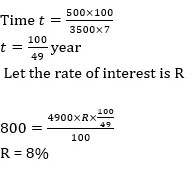# Quant Quiz for IBPS PO & IBPS CLERK PRE

Quant Quiz to improve your Quantitative Aptitude for SBI Po & SBI clerk exam IBPS PO Reasoning , IBPS Clerk Reasoning , IBPS RRB Reasoning, LIC AAO ,  and other competitive exams.

Q1. Each of the following question is followed by two quantities I, and II. You have to determine the value of the quantities using the information provided and accordingly compare the quantities. Mark your answer as per the instruction set provided below.
Quantity I: Time taken by Prabhas to cover a distance of 200 km (without stoppage) by car through which he covers a distance of 100 km in 1 hour when he stops to get fuel for 10 minutes.
Quantity II: Time taken by Nagarjuna to travel 20 km downstream if speed of boat and speed of stream be 7 kmph & 3 kmph respectively.
1. Quantity I>Quantity II
2. Quantity I≥Quantity II
3. Quantity I<Quantity II
4. Quantity I≤Quantity II
5. Quantity I=Quantity II or no relation
Q1. Ans(3)Q2. Each of the following question is followed by two quantities I, and II. You have to determine the value of the quantities using the information provided and accordingly compare the quantities. Mark your answer as per the instruction set provided below.
There are 3 red and 5 blue balls in the urn.
Quantity I: the probability of drawing 1 red ball and 2 blue balls.
Quantity II: the probability of drawing 2 red balls and 1 blue ball
1. Quantity I>Quantity II
2. Quantity I≥Quantity II
3. Quantity I<Quantity II
4. Quantity I≤Quantity II
5. Quantity I=Quantity II or no relation
Q2. Ans(1)Q3. Each of the following question is followed by two quantities I, and II. You have to determine the value of the quantities using the information provided and accordingly compare the quantities. Mark your answer as per the instruction set provided below.
In how many ways can 4 boys or 5 girls be selected?
Quantity I: there are 20 persons (boys and girls only) in the group out of which 12 are boys.
Quantity I: the group comprises 10 boys and 10 girls.
1. Quantity I>Quantity II
2. Quantity I≥Quantity II
3. Quantity I<Quantity II
4. Quantity I≤Quantity II
5. Quantity I=Quantity II or no relation
Q3. Ans(1)Q4. Each of the following question is followed by two quantities I, and II. You have to determine the value of the quantities using the information provided and accordingly compare the quantities. Mark your answer as per the instruction set provided below.
Quantity I: The length & breadth of a rectangle of perimeter 48 cm are in ratio 5:3. Find Area?
Quantity II: 135
1. Quantity I>Quantity II
2. Quantity I≥Quantity II
3. Quantity I<Quantity II
4. Quantity I≤Quantity II
5. Quantity I=Quantity II or no relation
Q4. Ans(5)Q5. Each of the following question is followed by two quantities I, and II. You have to determine the value of the quantities using the information provided and accordingly compare the quantities. Mark your answer as per the instruction set provided below.1. Quantity I>Quantity II
2. Quantity I≥Quantity II
3. Quantity I<Quantity II
4. Quantity I≤Quantity II
5. Quantity I=Quantity II or no relation
Q5. Ans(5)Q6.  Rakesh borrows Rs. 3500 from a bank at SI. After three years he paid Rs. 1500 to the bank and at the end of 5 years from the date of borrowing he paid Rs. 2725 to the bank to settle the account. Find the rate of interest.

A) 10%

B) 5%

C) 2.5%

D) 3.5%

E) None of these

Q6. Ans(B)Q7. Ashok borrowed some money at simple interest at the rate of 6 per cent per annul for the first two years, at the rate of 9 per cent per annul for the next three years and at the rate of 14% per cent per annul for the period beyond five years. If he pays a total interest of Rs. 11400 at the end of 9 years how much money did he borrow ?

(a) Rs. 16,000

(b) Rs. 14,000

(c) Rs. 18,000

(d) Rs. 12,000

(e) None of these

Q7. Ans(d)Q8. On Rs 3500 invested at a simple interest rate 7 per cent per annum, Rs 500 is obtained as interest in certain years, In order to earn Rs 800 as interest on Rs 4900 in the same number of years, what should be the rate of simple interest?

(a) 9%

(b) 10%

(c) 12%

(d) 8%

(e) None of these

Q8. Ans(d)Q9. A person invested a certain amount at simple interest at the rate of 6 per cent per annul earning Rs. 900 as an interest at the end of three years. Had the interest been compounded every year, how much more interest would he have earned on the same amount with the same interest rate after three years ?

(a) Rs. 38.13

(b) Rs. 25.33

(c) Rs. 55.08

(d) Rs. 35.30

(e) None of these

Q9. Ans(c)Q10. If Rs.1000 be invested at interest rate of 5% and the interest be added to the principle every 10 years, then In how many years  it will amount to Rs. 2000 ?Q10. Ans(a)Best E-books for LIC AAO 2019 : Get PDF here

ambitiousbaba.com need your support to Grow

I challenge you will get Best Content in Our PDFs with Detail solutions and Latest Pattern

Memory Based Puzzle E-book | 2016-19 Exams Covered

Get PDF here

Caselet Data Interpretation 200 Questions

Get PDF here
Puzzle & Seating Arrangement E-Book for BANK PO MAINS (Vol-1)

Get PDF here

### ARITHMETIC DATA INTERPRETATION 2019 E-book

Get PDF here
The Banking Awareness 500 MCQs E-book| Bilingual (Hindi + English)

Get PDF here

High Level DATA INTERPRETATION Practice E-BOOK

Get PDF here

### How to Access on App:-

1. Go to Playstore search Ambitious Baba or Click here to Install App